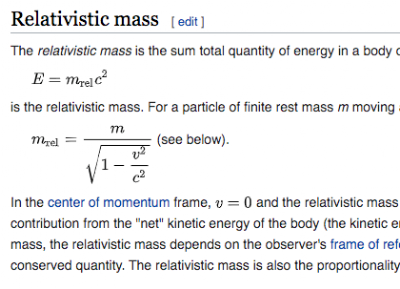## Friday, July 3, 2020

### Rest Mass

What is rest mass?

Rest mass is the mass of an object that is not moving.

We might be tempted to ask, "Why is this even a thing? How is this different from mass?"https://en.wikipedia.org/wiki/Mass_in_special_relativity(2020-07-03)
Very good question! Here's two reasons as to why rest mass is a thing.

First off, you probably have heard of the theory of relativity. Part of that includes the concept that mass changes as the velocity of the object increases. So, the mass of an object isn't a constant. It is a function of velocity.

Does that mean you feel heavier if you are riding in a car?

No. There's a part of the equation that divides the square of velocity of the thing moving by the square of the speed of light. That pretty much equals zero if you are driving around in a car.

302 / 186,0002 = not much

The second reason that rest mass is a thing is… it's complicated…

Electromagnetic energy, such as light, is considered to have momentum as long as it is moving. Momentum is a function of mass. So… Does that mean light has mass? If light has mass, problems arise and it makes people's heads hurt, so it is convenient to say light has momentum but not mass, which is a paradox of sorts.

Generally, the argument discussion, concept, paradox can be satisfied by saying that light has no rest mass, meaning that once it stops moving, it has no mass. Thus, the concept of rest mass is useful.

Because who want's there head to hurt?